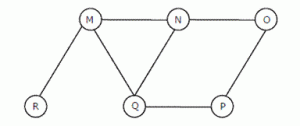# Data Structures and Algorithms | Set 14

• Difficulty Level : Medium
• Last Updated : 05 Jul, 2018

Following questions have been asked in GATE CS 2008 exam.

1. We have a binary heap on n elements and wish to insert n more elements (not necessarily one after another) into this heap. The total time required for this is
(A) Θ(logn)
(B) Θ(n)
(C) Θ(nlogn)
(D) Θ(n2)

Attention reader! Don’t stop learning now.  Practice GATE exam well before the actual exam with the subject-wise and overall quizzes available in GATE Test Series Course.

Learn all GATE CS concepts with Free Live Classes on our youtube channel.

The worst case time complexity for insertion in a binary heap is O(Logn) (Refer Wiki). So inserting n elements in a heap of size n should take Θ(nlogn) time.
But choice (B) seems to be more appropriate answer. One of the solution of O(n) complexity can be to take the ‘n’ elements of the heap and other ‘n’ elements together and construct heap in O(2n) = O(n). Thanks to pankaj for suggesting this solution.

2. The Breadth First Search algorithm has been implemented using the queue data structure. One possible order of visiting the nodes of the following graph is(A) MNOPQR
(B) NQMPOR
(C) QMNPRO
(D) QMNPOR

3. Consider the following functions:

```  f(n)   = 2^n
g(n)   = n!
h(n)   = n^logn ```

Which of the following statements about the asymptotic behaviour of f(n), g(n), and h(n) is true?
(A) f(n) = O(g(n)); g(n) = O(h(n))
(B) f(n) = Ω(g(n)); g(n) = O(h(n))
(C) g(n) = O(f(n)); h(n) = O(f(n))
(D) h(n) = O(f(n)); g(n) = Ω(f(n))

According to order of growth: h(n) < f(n) < g(n) (g(n) is asymptotically greater than f(n) and f(n) is asymptotically greater than h(n) ) We can easily see above order by taking logs of the given 3 functions

`   lognlogn < n < log(n!)  (logs of the given f(n), g(n) and h(n)).`

Note that log(n!) = Θ(nlogn)

4. The minimum number of comparisons required to determine if an integer appears more than n/2 times in a sorted array of n integers is

(A) Θ(n)
(B) Θ(logn)
(C) Θ(log*n)
(D) Θ(n)